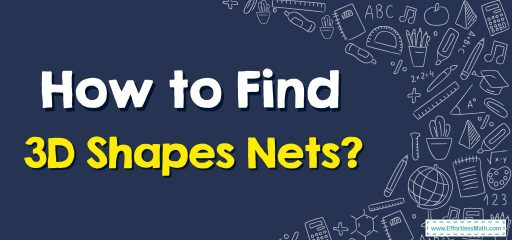# How to Find 3D Shapes Nets?

A net is an unfolded form of a 3D figure. In this step-by-step guide, you learn more about 3D shapes and finding 3D shape nets.Three-dimensional shapes are solids that are made up of three dimensions – length, breadth (width), and height. $$3D$$ in the word $$3D$$ shapes means three-dimensional.

## Step by step guide tofinding $$3D$$ shapes nets

$$3D$$ shapes are solid shapes or objects that have three dimensions (length, width, and height), unlike two-dimensional objects that have only length and width. Other important terms related to $$3D$$ geometric shapes are faces, edges, and vertices.

### $$3D$$ shapes nets

Using nets, we can understand three-dimensional shapes and their properties. A two-dimensional shape that can be folded to form a three-dimensional object is known as a geometric net. A solid may have different nets. In simple words, a net is an unfolded form of a $$3D$$ figure.

Here are some steps to determine if a net forms a solid:

1. Make sure that the solid and the net have the same number of faces and that the shape of the solid faces matches the shapes of the corresponding faces in the net.
2. Visualize how the net is to be folded to form the solid and make sure that all the sides fit together properly.

Nets are helpful when we need to find the surface area of the solids.

#### Cuboid

The cuboid is also known as a rectangular prism. The faces of the cuboid are rectangles. All angle measurements are $$90$$ degrees.

Take a matchbox, cut the edges, and flatten the box. This is the net for the cuboid. Now if you fold it back and glue it together as you open it, you get a cuboid.

#### Cube

The cube is defined as a three-dimensional square with $$6$$ equal sides. All faces of the cube have equal dimensions. Take a box of cheese cubes and cut it along the edges to make a net for a cube.

#### Cone

A cone is a solid object that has a circular base and also a vertex. It is a geometrical shape that tapers smoothly from the circular flat base to a point called the apex. Take a conical birth cap. When you cut a slit along its slope, you get a net for the cone.

#### Cylinder

A cylinder is a solid geometric shape that has two parallel circular bases connected by a curved surface. When you cut the curved surface of each cylindrical jar, you get a net for the cylinder. The net consists of two circles for the base and top and a rectangle for the curved surface.

#### Pyramid

A pyramid is also called a polyhedron. A pyramid can be a polygon, such as a square, triangle, and so on. It has three or more triangular faces that join together at a common point called the vertex. The net for a pyramid with a square base consists of a square with triangles along its four edges.

## Exercises for Finding $$3D$$ Shapes Nets

### What people say about "How to Find 3D Shapes Nets? - Effortless Math: We Help Students Learn to LOVE Mathematics"?

No one replied yet.

X
30% OFF

Limited time only!

Save Over 30%

SAVE $5 It was$16.99 now it is \$11.99